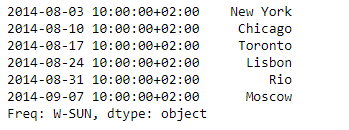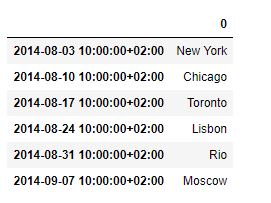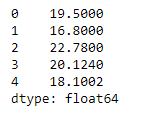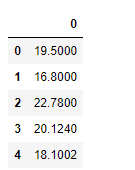# Pandas Series.to_frame()

## Python | Pandas Series.to_frame()

Pandas series is a One-dimensional ndarray with axis labels. The labels need not be unique but must be a hashable type. The object supports both integer- and label-based indexing and provides a host of methods for performing operations involving the index.

Pandas` Series.to_frame()` function is used to convert the given series object to a dataframe.

Syntax: Series.to_frame(name=None)

Parameter :
name : The passed name should substitute for the series name (if it has one).

Returns : data_frame : DataFrame

Example #1: Use `Series.to_frame()` function to convert the given series object to a dataframe.

 `# importing pandas as pd` `import` `pandas as pd` ` ` `# Creating the Series` `sr ``=` `pd.Series([``'New York'``, ``'Chicago'``, ``'Toronto'``, ``'Lisbon'``, ``'Rio'``, ``'Moscow'``])` ` ` `# Create the Datetime Index` `didx ``=` `pd.DatetimeIndex(start ``=``'2014-08-01 10:00'``, freq ``=``'W'``, ` `                     ``periods ``=` `6``, tz ``=` `'Europe/Berlin'``) ` ` ` `# set the index` `sr.index ``=` `didx` ` ` `# Print the series` `print``(sr)`

Output :Now we will use `Series.to_frame()` function to convert the given series object to a dataframe.

 `# convert to dataframe` `sr.to_frame()`

Output :As we can see in the output, the `Series.to_frame()` function has successfully converted the given series object to a dataframe.

Example #2: Use `Series.to_frame()` function to convert the given series object to a dataframe.

 `# importing pandas as pd` `import` `pandas as pd` ` ` `# Creating the Series` `sr ``=` `pd.Series([``19.5``, ``16.8``, ``22.78``, ``20.124``, ``18.1002``])` ` ` `# Print the series` `print``(sr)`

Output :Now we will use `Series.to_frame()` function to convert the given series object to a dataframe.

 `# convert to dataframe` `sr.to_frame()`

Output :As we can see in the output, the `Series.to_frame()` function has successfully converted the given series object to a dataframe.

Last Updated on March 17, 2022 by admin

## Python – Split list into lists by particular valuePython – Split list into lists by particular value

Python | Split list into lists by particular value The splitting of lists is quite

## Python – Find all duplicate characters in stringPython – Find all duplicate characters in string

Python – Find all duplicate characters in string Given a string, find all the duplicate

## How to Convert Int to Bytes in Python?How to Convert Int to Bytes in Python?

How to Convert Int to Bytes in Python? An int object can be used to

Reading CSV files in Python A CSV (Comma Separated Values) file is a form of plain text document

## How to get file size in Python?How to get file size in Python?

How to get file size in Python? We can follow different approaches to get the

## Python – Draw Star Using Turtle GraphicsPython – Draw Star Using Turtle Graphics

Python – Draw Star Using Turtle Graphics In this article, we will learn how to

## Remove all the occurrences of an element from a list in PythonRemove all the occurrences of an element from a list in Python

Remove all the occurrences of an element from a list in Python The task is